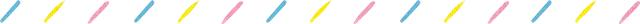# 信号与系统，信号与系统

### 信号与系统

1. 用MATLAB画出正弦离散序列的时域波形。

N=100;

n=-N:N;

w0=0.2;

f1=cos((pi*n*w0)/8);

f2=cos(2*n*w0);

subplot(211);

stem(n,f1); grid on;

title('f1=cos((pi*n*w0)/8)');

xlabel('n'); ylabel('f1(n)');

subplot(212);

stem(n,f2); grid on;

title('f2=cos(2*n)');

xlabel('n'); ylabel('f2(n)');

2.已知信号

，画出

t=-20:0.01:20;

f1=0.25*(t+1).*(t>-4&t<0)+1.*(t>0&t<2)+0.*(t>=2&t<=-4&t==0);

subplot(211);

plot(t,f1); grid on;

title('f1=(t+1)/4.*(t>-4&t<0)+1.*(t>0&t<2)+0.*(t>=2&t<=-4)');

xlabel('t'); ylabel('f(t)');

%f2=0.25*((-2)*t+5).*(t>4&t<12)+1.*(t>0&t<4)+0.*(t>=12&t<=0&t==4);

f2=-0.25*(t+1).*(t>2&t<4)+1.*(t>1&t<2)+0.*(t>=4&t<=1&t==2);

subplot(212);

plot(t,f2); grid on;

title('f2=0.25*(-2*t+5).*(t>-4&t<0)+1.*(t>0&t<2)+0.*(t>=2&t<=-4&t==0)');

xlabel('t'); ylabel('f(-2t+4)');

3、 已知描述系统的微分方程和激励信号e(t) 分别如下，试用解析方法求系统的单位冲激响应h(t)和零状态响应r(t)，并用MATLAB绘出系统单位冲激响应和系统零状态响应的波形。

a=[1 4 4];

b=[1 3];

subplot(211)

impulse(b,a,4); %冲激响应函数

title('ÏµÍ³µ¥Î»³å¼¤ÏìÓ¦');

c=[1 4 4];

d=[1 3];

p1=0.001;

t=0:p1:10;

x1=exp(-t);

subplot(212)

%lsim(b,a,x1,t);

lsim(d,c,x1,t);

title('ÏµÍ³Áã×´Ì¬ÏìÓ¦');

1、如下图所示的电路中，已知

，且两电感上初始电流分别为

，如果以电阻

（v）作用下的全响应。

A=[-8 4;4 -8];

B=[1;0];

C=[4,-4];

D=;

x0=[2;0];

t=0:0.01:10; %控制坐标

E=[12.*ones(size(t))];

[r,x]=lsim(A,B,C,D,E,t,x0);

plot(t,r);

2、编写代码实现一个频率50Hz，占空比为50%和25%的周期矩形波（方波）信号。

t = -0.065:0.0001:0.065;

y1=square(2*pi*50*t,50);

subplot(211)

plot(t,y1);

axis([-0.0625 0.0625 -1.5 1.5]);

grid on;

y2=square(2*pi*50*t,25);

subplot(212)

plot(t,y2);

axis([-0.0625 0.0625 -1.5 1.5]);

grid on;

### 20成电通信 | 信号与系统，我们将重点帮你整理好了 1.1 绪论 1.2 信号的描述和分类 一.连续时间和离散时间 二.信号能量与功率 三.自变量的变换 公众号更多阅读:T学长 以初试总分380分的成绩顺利考入电子科技大学信息与通信工程学院。其中专业课141分，在复试的650多人中排名前十。深入研究通信与系统专业课的知识难点及高频考点，并总结多种学习技巧，可以给大家节约复习时间、帮助建立学科框架、提升综合运用知识能力。本人熟悉信通院的各类信息动态，希望可以帮到学弟学妹们，期待与你们电子科技大学见！

1.1 绪论

1.2 信号的描述和分类

1、数学描述：使用具体的数学表达式，把信号描述为一个或若干个自变量的函数或序列的形式。

2、波形描述：按照函数自变量的变化关系，把信号的波形画出来。 “信号”与“函数”两词常相互通用。[例1.2.1] 判断下列信号是否为周期信号，若是，确定其周期。

（1）f1(t) = sin2t + cos3t （2）f2(t) = cos2t + sinπt 解：

（1） sin2t 是周期信号，其角频率和周期分别为 ω1= 2 rad/s ， T1= 2π/ ω1= πs cos3t 是周期信号，其角频率和周期分别为 ω2= 3 rad/s ， T2= 2π/ ω2= (2π/3) s 由于T1/T2= 3/2为有理数，故f1(t)为周期信号， 其周期为T1和T2的最小公倍数2π。

（2） cos2t 和sinπt的周期分别为T1= πs， T2= 2 s， 由于T1/T2为无理数，故f2(t)为非周期信号。

1：时移。在离散时间信号上表示成x[n]和进行时移了的x[n-n0]（n0为常数），这两个信号在形状上是完全一样的，但是在位置上互相有一个移位，在连续时间信号域内有x（t）和进行时移了的x（t-t0）（t0为常数），这里的x（t-t0）代表一个延时为t0（若t0为正）的x（t），或者代表一个超前为t0（若t0为负）的x（t），表示时间上的平移，或超前，或延迟，在形状上没有变化。但是要注意的是无论自变量t前边的系数是否为1，都是对于时间t而言的时移，对于离散时间信号也是一样。

2. 尺度变换（横坐标展缩） 将f (t) → f (a t) ， 称为对信号f (t)的尺度变换。若a >1 ，则波形沿横坐标压缩；若0< a < 1 ，则展开。如 （1） a > 1 则 f (at)将 f (t)的波形沿时间轴压缩至原来的1/a 对于离散信号，由于f (a k) 仅在为a k 为整数时才有意义， 进行尺度变换时可能会使部分信号丢失。因此一般不作波形的尺度变换。20成电通信考研 | 恭喜你，在考研之前看到了这篇靠谱的分析！

20届通信|电科通信与信息系统初试攻略！

20届通信|学长为你讲解信号与系统第二讲！